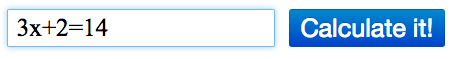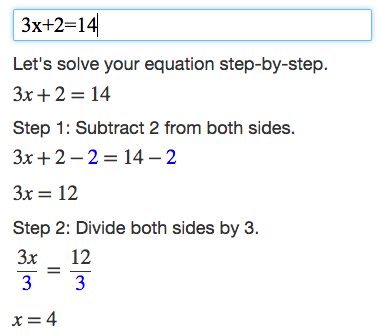Algebra Calculator

Step-by-step calculator that understands algebra.

Quick Tutorial

This is a quick tutorial for the Algebra Calculator, a step-by-step calculator for algebra.

How to Use the Calculator

To use the calculator, you enter the math problem that you want to calculate into the text box.For example, if you enter the equation 3x+2=14 into the text box, the calculator will print a step-by-step explanation of how to solve 3x+2=14.Examples

Trying the examples on the Examples page is the quickest way to learn how to use the calculator.

Math Symbols

If you would like to create your own math expressions, here are some symbols that the calculator understands: